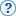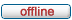# forum.alglib.net

ALGLIB forum
 It is currently Wed Jun 29, 2022 1:22 pm

 All times are UTC

### Forum rules

1. This forum can be used for discussion of both ALGLIB-related and general numerical analysis questions
2. This forum is English-only - postings in other languages will be removed.

 Print view Previous topic | Next topic
Author MessagePost subject: Using MinLM to find parameters to a 5PL cuverPosted: Mon Sep 13, 2010 4:17 pmJoined: Mon Sep 13, 2010 3:27 pm
Posts: 5
This is my first time using AlgLib.

I am currently using solver in excel to give me the parameters to a 5PL curve. I have a formula that I plug in the parameters to redraw a calculated curve to match the observed curve. I am not sure of the Levenberg-Marquardt is the best way of doing this but I need to be able to test the parameters without using solver. I'm not even sure how to plug in the parameters or the formula into functions. The basic formula for a 5PL curve is 1+((a-d)/(1+(x/c)^b)^m) and the parameters are A,B,C,D,M. A is lowest point in the curve, D is the highest point in the curve, B is midpoint, C is the maximum reading at infinite and m is the assymetry value.

Thank you.

TopPost subject: Re: Using MinLM to find parameters to a 5PL cuverPosted: Mon Sep 13, 2010 7:42 pmSite Admin

Joined: Fri May 07, 2010 7:06 am
Posts: 871
Take a look at the example below. It is from development version of ALGLIB which is somewhere between 2.6 and 3.0, but it should be compatible with ALGLIB 2.6 (you may nned to change one or two function names, and that's all). The main idea is that you initialize fitting object, and then repeatedly call LSFitNonlinearIteration(), updating function value and/or gradient (depending on what is requested by algorithm).

Unfortunately, this example is not distributed with ALGLIB for VBA, because all my example are console-oriented, and VBA does not supports console, so you can't compile it (it contains calls to ConsoleOutputString() function, which is not provided by VBA).

Code:
Public Sub DemoRoutine()
Dim M As Long
Dim N As Long
Dim K As Long
Dim Y() As Double
Dim X() As Double
Dim C() As Double
Dim Rep As LSFitReport
Dim State As LSFitState
Dim Info As Long
Dim EpsF As Double
Dim EpsX As Double
Dim MaxIts As Long
Dim I As Long
Dim J As Long
Dim A As Double
Dim B As Double

ConsoleOutputString  "Fitting 0.5(1+cos(x)) on [-pi,+pi] with exp(-alpha*x^2)" _
&  vbNewLine

'
' Fitting 0.5(1+cos(x)) on [-pi,+pi] with Gaussian exp(-alpha*x^2):
' * without Hessian (gradient only)
' * using alpha=1 as initial value
' * using 1000 uniformly distributed points to fit to
'
' Notes:
' * N - number of points
' * M - dimension of space where points reside
' * K - number of parameters being fitted
'
N = 1000#
M = 1#
K = 1#
A = -Pi()
B = +Pi()

'
'
ReDim Y(0 To N-1)
ReDim X(0 To N-1, 0 To M-1)
ReDim C(0 To K-1)
For I=0# To N-1# Step 1
X(I,0#) = A+(B-A)*I/(N-1#)
Y(I) = 0.5*(1#+Cos(X(I,0#)))
Next I
C(0#) = 1.0
EpsF = 0.0
EpsX = 0.0001
MaxIts = 0#

'
' Solve
'
Call LSFitNonlinearFG(X, Y, C, N, M, K, True, State)
Call LSFitNonlinearSetCond(State, EpsF, EpsX, MaxIts)
Do While LSFitNonlinearIteration(State)
If State.NeedF then

'
' F(x) = Exp(-alpha*x^2)
'
State.F = Exp(-(State.C(0#)*Square(State.X(0#))))
End If
If State.NeedFG then

'
' F(x)      = Exp(-alpha*x^2)
' dF/dAlpha = (-x^2)*Exp(-alpha*x^2)
'
State.F = Exp(-(State.C(0#)*Square(State.X(0#))))
State.G(0#) = -(Square(State.X(0#))*State.F)
End If
Loop
Call LSFitNonlinearResults(State, Info, C, Rep)
ConsoleOutputString  "alpha:   " &  FormatFReal(C(0#), 0, 3) _
&  vbNewLine
ConsoleOutputString  "rms.err: " &  FormatFReal(Rep.RMSError, 0, 3) _
&  vbNewLine
ConsoleOutputString  "max.err: " &  FormatFReal(Rep.MaxError, 0, 3) _
&  vbNewLine
ConsoleOutputString  "Termination type: " &  FormatInteger(Info, 0) _
&  vbNewLine
ConsoleOutputString  vbNewLine &  vbNewLine
End Sub

TopPost subject: Re: Using MinLM to find parameters to a 5PL cuverPosted: Tue Sep 14, 2010 7:44 pmJoined: Mon Sep 13, 2010 3:27 pm
Posts: 5
Sergy, in the example that you sent do I need to include a formula for the degrees of freedom? Or do I just have to have it repeat the formula until it gets to the minimum?

TopPost subject: Re: Using MinLM to find parameters to a 5PL cuverPosted: Wed Sep 15, 2010 7:41 pmSite Admin

Joined: Fri May 07, 2010 7:06 am
Posts: 871
Degrees of freedom? I don't understand it.

You have to repeatedly calculate it until it converges to minimum (while loop will end)

TopPost subject: Re: Using MinLM to find parameters to a 5PL cuverPosted: Wed Sep 15, 2010 8:01 pmJoined: Mon Sep 13, 2010 3:27 pm
Posts: 5
I guess I was confused by the comment line where you show dF/dAlpha

TopPost subject: Re: Using MinLM to find parameters to a 5PL cuverPosted: Fri Sep 17, 2010 1:13 pmJoined: Mon Sep 13, 2010 3:27 pm
Posts: 5
Still having issues. Does not seem to be giving me a change in parameters or finding a minimum. Here is my code.

Private Sub test22()
Dim x() As Double
Dim Y(7) As Double
Dim C() As Double
Dim M As Long
Dim N As Long
Dim K As Long
Dim I As Integer
Dim info As Long
Dim EpsF As Double
Dim EpsX As Double
Dim MaxIts As Double
Dim Rep As lsfit.LSFitReport
Dim State As lsfit.LSFitState

' n = number of points in the curve
' m = dimension of space points reside
' k = number of parameters

M = 8
N = 8
K = 5

ReDim x(N, M)
ReDim C(K)

Y(0) = 1.8796
Y(1) = 1.0027
Y(2) = 0.5224
Y(3) = 0.2774
Y(4) = 0.1487
Y(5) = 0.0896
Y(6) = 0.0625
Y(7) = 0.0222

x(0, 0) = 8
x(1, 0) = 4
x(2, 0) = 2
x(3, 0) = 1
x(4, 0) = 0.5
x(5, 0) = 0.25
x(6, 0) = 0.125
x(7, 0) = 0

C(0) = 0.022 'e3
C(1) = 1# 'e4
C(2) = 1.5 'e5
C(3) = 10 'e6
C(4) = 0.35 'e7

EpsF = 0
EpsX = 0.0001
MaxIts = 10

Call LSFitNonlinearFGH(x, Y, C, N, M, K, State)
Call LSFitNonlinearSetCond(State, EpsF, EpsX, MaxIts)

Do While LSFitNonlinearIteration(State)

If (State.NeedF) Then

State.F = (1 / 6.33679999999973E-04) * ((Y(0) - (((State.C(0) - State.C(3)) / (1 + (x(0, 0) / State.C(2)) ^ State.C(1)) ^ State.C(4)) + State.C(3))) ^ 2) / 3 + _
(1 / 3.12049999999964E-03) * ((Y(1) - (((State.C(0) - State.C(3)) / (1 + (x(1, 0) / State.C(2)) ^ State.C(1)) ^ State.C(4)) + State.C(3))) ^ 2) / 3 + _
(1 / 1.41120000000106E-04) * ((Y(2) - (((State.C(0) - State.C(3)) / (1 + (x(2, 0) / State.C(2)) ^ State.C(1)) ^ State.C(4)) + State.C(3))) ^ 2) / 3 + _
(1 / 1.18580000000007E-04) * ((Y(3) - (((State.C(0) - State.C(3)) / (1 + (x(3, 0) / State.C(2)) ^ State.C(1)) ^ State.C(4)) + State.C(3))) ^ 2) / 3 + _
(1 / 7.19999999999957E-53) * ((Y(4) - (((State.C(0) - State.C(3)) / (1 + (x(4, 0) / State.C(2)) ^ State.C(1)) ^ State.C(4)) + State.C(3))) ^ 2) / 3 + _
(1 / 5.61800000000029E-53) * ((Y(5) - (((State.C(0) - State.C(3)) / (1 + (x(5, 0) / State.C(2)) ^ State.C(1)) ^ State.C(4)) + State.C(3))) ^ 2) / 3 + _
(1 / 1.96020000000002E-04) * ((Y(6) - (((State.C(0) - State.C(3)) / (1 + (x(6, 0) / State.C(2)) ^ State.C(1)) ^ State.C(4)) + State.C(3))) ^ 2) / 3 + _
(1 / 4.99999999999994E-93) * ((Y(7) - (((State.C(0) - State.C(3)) / (1 + (x(7, 0) / State.C(2)) ^ State.C(1)) ^ State.C(4)) + State.C(3))) ^ 2) / 3

End If

If (State.NeedFG) Then

State.F = (1 / 6.33679999999973E-04) * ((Y(0) - (((State.C(0) - State.C(3)) / (1 + (x(0, 0) / State.C(2)) ^ State.C(1)) ^ State.C(4)) + State.C(3))) ^ 2) / 3 + _
(1 / 3.12049999999964E-03) * ((Y(1) - (((State.C(0) - State.C(3)) / (1 + (x(1, 0) / State.C(2)) ^ State.C(1)) ^ State.C(4)) + State.C(3))) ^ 2) / 3 + _
(1 / 1.41120000000106E-04) * ((Y(2) - (((State.C(0) - State.C(3)) / (1 + (x(2, 0) / State.C(2)) ^ State.C(1)) ^ State.C(4)) + State.C(3))) ^ 2) / 3 + _
(1 / 1.18580000000007E-04) * ((Y(3) - (((State.C(0) - State.C(3)) / (1 + (x(3, 0) / State.C(2)) ^ State.C(1)) ^ State.C(4)) + State.C(3))) ^ 2) / 3 + _
(1 / 7.19999999999957E-53) * ((Y(4) - (((State.C(0) - State.C(3)) / (1 + (x(4, 0) / State.C(2)) ^ State.C(1)) ^ State.C(4)) + State.C(3))) ^ 2) / 3 + _
(1 / 5.61800000000029E-53) * ((Y(5) - (((State.C(0) - State.C(3)) / (1 + (x(5, 0) / State.C(2)) ^ State.C(1)) ^ State.C(4)) + State.C(3))) ^ 2) / 3 + _
(1 / 1.96020000000002E-04) * ((Y(6) - (((State.C(0) - State.C(3)) / (1 + (x(6, 0) / State.C(2)) ^ State.C(1)) ^ State.C(4)) + State.C(3))) ^ 2) / 3 + _
(1 / 4.99999999999994E-93) * ((Y(7) - (((State.C(0) - State.C(3)) / (1 + (x(7, 0) / State.C(2)) ^ State.C(1)) ^ State.C(4)) + State.C(3))) ^ 2) / 3
End If

Loop

Call LSFitNonlinearResults(State, info, C, Rep)

Debug.Print State.C(0), State.C(1), State.C(2), State.C(3), State.C(4)
Debug.Print C(0), C(1), C(2), C(3), C(4)
End Sub

TopPost subject: Re: Using MinLM to find parameters to a 5PL cuverPosted: Mon Sep 20, 2010 2:56 pmJoined: Mon Sep 13, 2010 3:27 pm
Posts: 5
Are the values in c() supposed to be changed through the lsFit functions. Everytime I run the function the original c() values are always returned even though the function iterates 32 times I don't see any change in the values.

TopPost subject: Re: Using MinLM to find parameters to a 5PL cuverPosted: Mon Sep 20, 2010 4:47 pmSite Admin

Joined: Fri May 07, 2010 7:06 am
Posts: 871
I see two errors in your code:
1. you don't calculate gradient value on State.NeedFG, function assumes that it is zero, that's why nothing changes
2. you should not calculate function/gradient for all points at once. On return from LSFitNonlinearIteration(State) you have point coordinates stored in State.X and function parameters stored in State.C. You should calculate function/gradient for one and only one point - which is specified by State.X - and call LSFitNonlinearIteration() again. It will cycle through points automatically

TopDisplay posts from previous: All posts1 day7 days2 weeks1 month3 months6 months1 year Sort by AuthorPost timeSubject AscendingDescending

 All times are UTC

#### Who is online

Users browsing this forum: No registered users and 3 guests

 You cannot post new topics in this forumYou cannot reply to topics in this forumYou cannot edit your posts in this forumYou cannot delete your posts in this forumYou cannot post attachments in this forum

Search for: